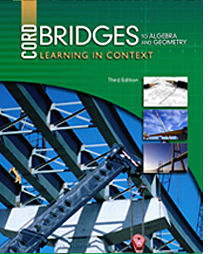# Bridges to Algebra and Geometry - 3rd Edition

## Chapter 8: Powers and Roots

Some links are repeated for use with more than one lesson.

### 8.1 Exponents and Powers

http://www.mathsisfun.com/definitions/exponent.html

http://www.regentsprep.org/Regents/math/ALGEBRA/Ao5/LExp.htm
Another page that gives the basic definition for exponents.

http://www.coolmath.com/prealgebra/05-order-of-operations/04-order-of-operations-exponents-EMDAS-01.htm
This series of pages presents how exponents fit into the order of operations for expressions, with examples.

http://www.regentsprep.org/regents/math/algebra/aop2/indexAOP2.htm
Review and practice the topic of order of operations, including exponents.

http://www.sosmath.com/algebra/logs/log3/log3.html
This site lists the exponent rules.

### 8.2 Properties of Exponents

http://www.mathsisfun.com/algebra/exponent-laws.html
This page defines the rules for working with exponents, including negative and fractional exponents, with practice problems.

http://www.algebralab.org/lessons/lesson.aspx?file=Algebra_ExponentsRules.xml
This page lists the rules for handling exponents, including examples and practice problems.

http://usablealgebra.landmark.edu/algebra/exponents/rules.php
This page gives an complete and concise summary of the properties of exponents with examples.

http://www.math.com/school/subject1/lessons/S1U1L8GL.html
This series of pages defines the rules for working with exponents, including some common mistakes to avoid, and some practice.

http://www.sosmath.com/algebra/logs/log3/log3.html
This site lists the exponent rules.

### 8.3 Scientific Notation

http://janus.astro.umd.edu/astro/scinote/
This site shows examples of how to change from scientific notation to normal numbers and vice versa and allows the student to practice these concepts.

http://www.regentsprep.org/regents/math/algebra/ao2/indexAO2.htm
This site includes several pages explaining and operating with numbers in scientific notation.

http://www.mathsisfun.com/numbers/scientific-notation.html
This page explains scientific and engineering notation, and includes some practice questions.

### 8.4 The Metric System

http://www.worldwidemetric.com/metcal.htm
This site has a metric conversion calculator.

http://www.mathsisfun.com/measure/metric-system.html
This page is an introduction to the metric system and its common prefixes applied to various real-world measures, including practice problems.

### 8.5 Square Roots and Irrational Numbers

http://www.mathsisfun.com/square-root.html
This page relates squares and square roots, and demonstrates how to approximate square roots, including practice problems.

http://www.squarerootcalculator.co
This page includes a simple square root calculator, and also advice for calculating square roots with various scientific calculators.

http://www.mathsisfun.com/irrational-numbers.html
This page defines irrational numbers, including some famous irrational numbers and practice problems

http://www.math.com/school/subject1/lessons/S1U1L9GL.html
This site explains how to find the square roots of numbers.